##### Question

In: Mechanical Engineering

# Determine the critical resolved shear stress for an iron crystal which deforms by simultaneous slip on...

Determine the critical resolved shear stress for an iron crystal which deforms by simultaneous slip on (110) , (110) , (110), and (110)  when the tensile stress along  is 95.2 MPa. {Manual calculations perfectly OK. But it would be good to do this on a spreadsheet.}

## Solutions

##### Expert Solution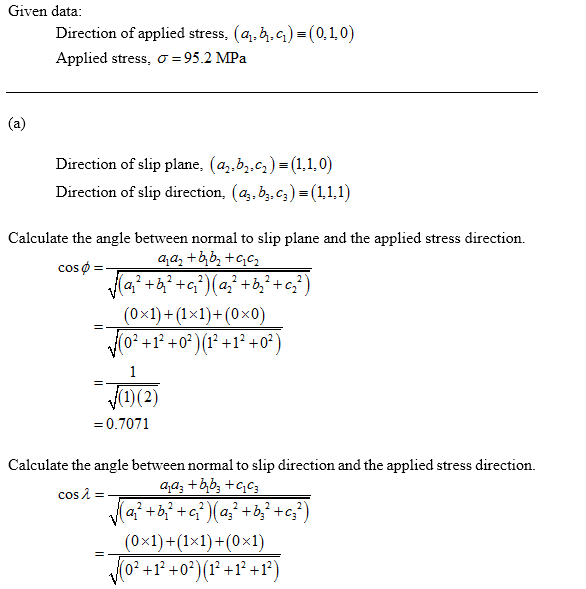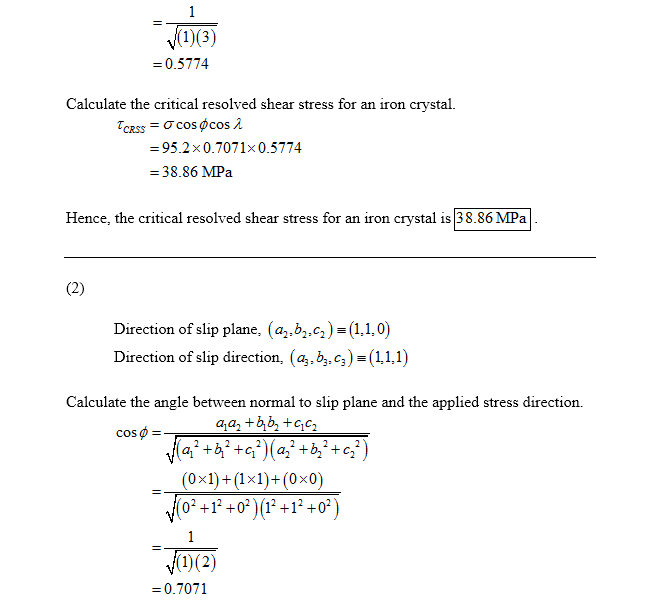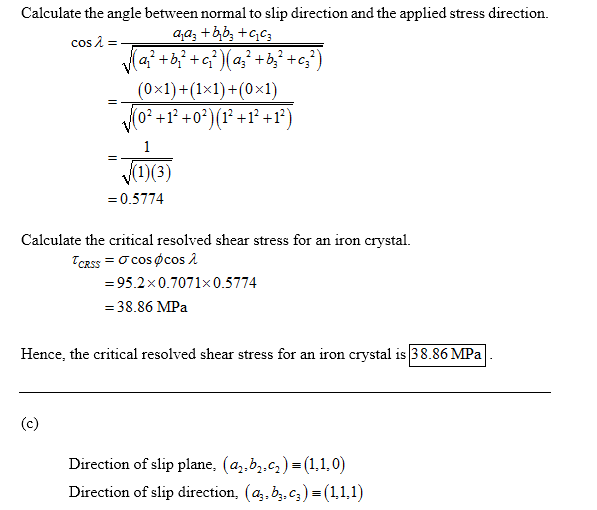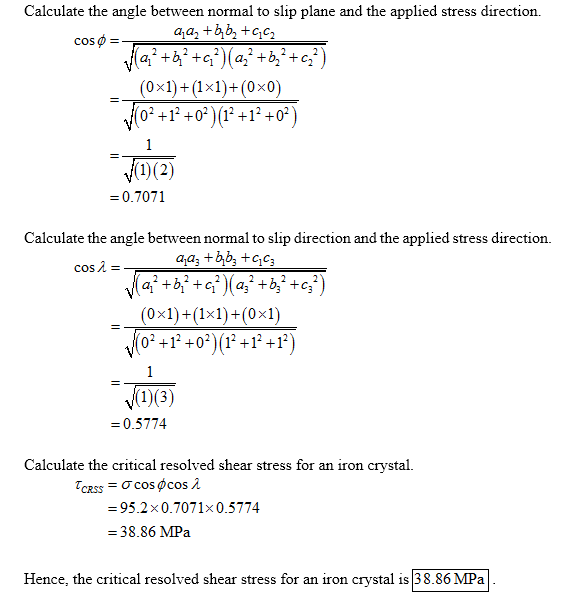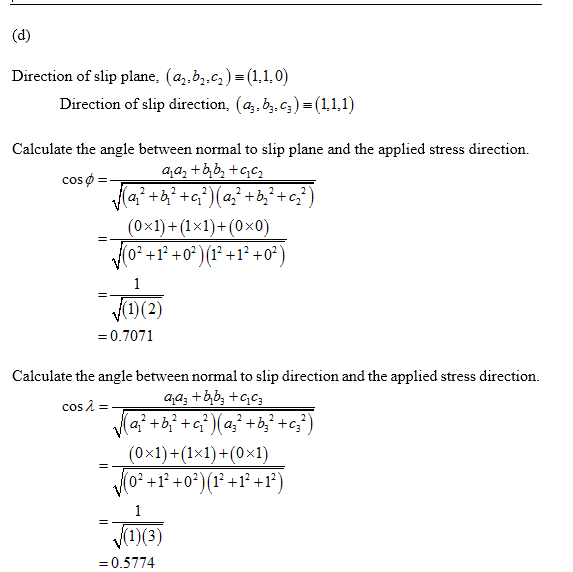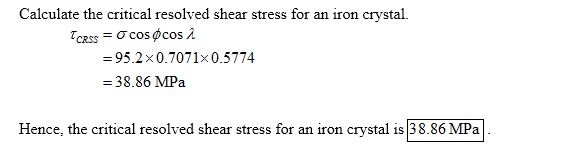## Related Solutions

##### (c) Draw a schematic graph of resolved shear stress versus shear strain for a single crystal...
(c) Draw a schematic graph of resolved shear stress versus shear strain for a single crystal of a pure metal. Clearly label the point of the critical resolved shear stress and the three subsequent stages of dislocation behaviour. Describe these three stages. (d) Explain why the critical resolved shear stress is much lower than the theoretical strength, based on the force required to break atomic bonds within the structure.
##### Assume if the shear stress in steel exceeds about 4.00x108 N/m2 the steel   ruptures. (a) Determine...
Assume if the shear stress in steel exceeds about 4.00x108 N/m2 the steel   ruptures. (a) Determine the shearing force necessary to shear a steel bolt 1.25 cm in diameter. (b) Determine the shearing force necessary to punch a 1.60-cm-diameter hole in a steel plate 0.530 cm thick
##### The beam is supported by a pin at A and a short link BC. If P = 10kN , determine the average shear stress developed...
The beam is supported by a pin at A and a short link BC.If P = 10kN, determine the average shear stress developed in the pins atA, B, and C . All pins are in double shear as shown, and each has a diameter of 17mm
##### Determine the shear stress developed on a 3-m circular shaft with D=250 mm, G = 83GPa...
Determine the shear stress developed on a 3-m circular shaft with D=250 mm, G = 83GPa applied with the torque, T = 100 kN-m, a. at the center of the cross-section b. at a point 50 mm away from the center c. at a point 100 mm away from the center d. maximum distance from the center
##### The beam is constructed from three boards. (Figure 1) The allowable shear stress for the wood is τallow=335 psi. Each nail can resist a shear force of 395 lb. Part A Determine the maximum loads P tha...
The beam is constructed from three boards. (Figure 1) The allowable shear stress for the wood is τallow=335 psi. Each nail can resist a shear force of 395 lb.Part ADetermine the maximum loads P that it can support.Express your answer to three significant figures and include appropriate units.Part BWhat is the maximum allowable spacing s of the nails used to hold the top flange to the web? (Consider only regions AC and BD.)Express your answer to three significant figures and...
##### Establish SABMILLER'S distinctive resources/core competences and determine the extent to which they match its critical success...
Establish SABMILLER'S distinctive resources/core competences and determine the extent to which they match its critical success factors